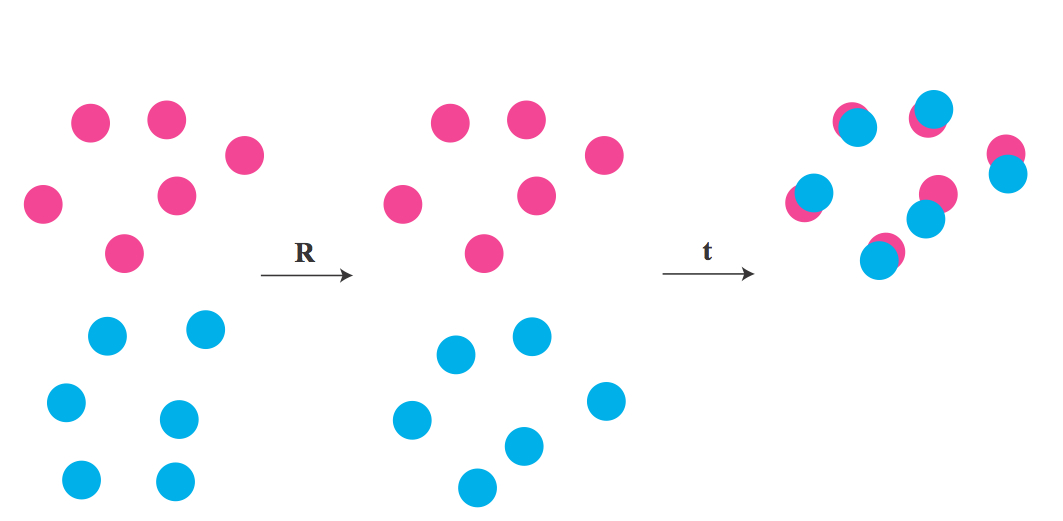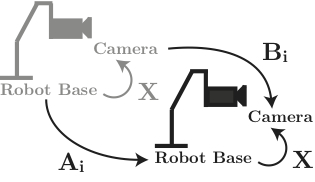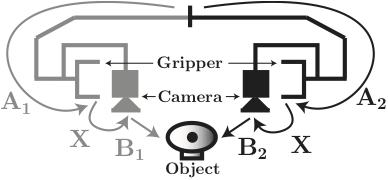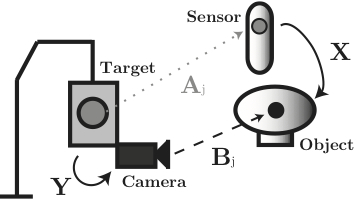Calibration and Registration Techniques for Robotics

Please note I have taken a position at The Cooper Union. Please visit my Cooper webpage.

Calibration and registration techniques are important tools in robotics. There have been many techniques that have been developed which include both closed form solutions and iterative solutions. This website will concentrate on closed form solutions that are commonly used in robotics. Though many of the following solutions have weighted solutions (that weight certain points more than others), this website will concentrate on unweighted closed form solutions. The solutions will be presented as m-files suitable for MATLAB. For a review of many of these algorithms, please check out

M. Shah, R. D. Eastman, T. Hong, An Overview of Robot-Sensor Calibration Methods for Evaluation of Perception Systems, Performance Metrics for Intelligent Systems, (2012).

I would be interested in hearing from you if you found these algorithms useful, find any bugs, or have any suggestions, questions, or problems. Please email me at "mili at cooper dot edu."

We will split these techniques into three categories:
* Registering Two Sets of 3DoF Data
* Registering Two Sets of 6DoF Data with 1 Unknown
* Registering Two Sets of 6DoF Data with 2 Unknowns

Registering Two Sets of 3DoF Data

Probably the most simple registration problem involves registering two sets of 3 degrees of freedom (DoF) data. This type of data typically involves positional data composed of $x,y, z$ values. However, many of the techniques that follow can be adapted to higher (and lower) dimensional data easily. Here we assume that the 3DoF data from two systems are stored in matrices $\mathbf{A}=[a_1, a_2, ... ,a_n]$ and $\mathbf{B}=[b_1, b_2, ..., b_n]$ where
$b_i = \mathbf{R}a_i + \mathbf{t}$
Here the unknown $\mathbf{R}$ is a 3x3 rotation matrix and the unknown $\mathbf{t}$ is a 3D translation vector. For this setup, we are trying to rotate and translate the data in $\mathbf{A}$ to line up with the data in $\mathbf{B}$.For example, in the picture above we have a set of blue dots $b_i$ and pink dots $a_i$ and we can see we have found a rotation $\mathbf{R}$ and translation $\mathbf{t}$ that best lines the points up. Mathematically we can express this as an optimization problem $\min_{\mathbf{R},\mathbf{t}}\sum_{i=1}^n\|\mathbf{R}a_i + \mathbf{t}-b_i\|$ which basically minimizes the distance between the transformed points $a_i$ and $b_i$. There have been many closed form solutions to this problem which include:

• K.S. Arun, T.S. Huang, S.D. Blostein, Least-squares fitting of two 3-d point sets, IEEE Trans. Pattern Anal. Mach. Intell. 9 (5) (1987) 698-700. code
• B.K.P. Horn, H.M. Hilden, S. Negahdaripour, Closed-form solution of absolute orientation using orthonormal matrices, J. Opt. Soc. Am. A 5 (1988) 1127-1135. code
• B.K.P. Horn, Closed-form solution of absolute orientation using unit quaternions, J. Opt. Soc. Am. A 4 (1987) 629-642. code
• M.W. Walker, L. Shao, R.A. Volz, Estimating 3-d location parameters using dual number quaternions, CVGIP: Image Underst. 54 (3) (1991) 358-367. code
• S. Umeyama, Least-squares estimation of transformation parameters between two point patterns, IEEE Trans. Pattern Anal. Mach. Intell. 13 (4) (1991) 376- 380. code

Code has been included at the end of each reference. The first algorithm by Arun, Huang, and Blostein is probably the most famous. However, the formulation of this method requires $\mathbf R$ just to be an orthogonal matrix without enforcing $\textrm{det}( \mathbf{R})=1$. Thus, the $\mathbf{R}$ that the algorithm spits out may not be an actual rotation but instead a reflection. As a consequence, one has to be cautious when using this method. A similar problem comes around with the second algorithm by Horn. This methods takes into consideration the polar decomposition of a matrix. But again it does not require that $\textrm{det}( \mathbf{R})=1$; thus there may be problems. The third algorithm again by Horn uses quaternions and thus you do not have to worry about calculating reflections for $\mathbf R$ instead of rotations. However, in this algorithm there may be a problem with the solution since the derivation of the rotation representation for a quaternion is not unique. All the methods mentioned so far use the rotation $\mathbf R$ to to solve for the translation $\mathbf t$. A different formulation can be found in the fourth algorithm. This methods claims to be faster than the previous methods and is supported by the conclusions found in

• Eggert, David W., Adele Lorusso, and Robert B. Fisher. "Estimating 3-D rigid body transformations: a comparison of four major algorithms." Machine Vision and Applications 9.5-6 (1997): 272-290.

for large n but slowest for small n. However, this work found that the Horn method (second algorithm) is actually fastest for small n and slowest for large n. In either case, the first algorithm was actually the second fastest. In addition, with regards to stability, they found that the first algorithm to be most stable. As a result, we claim the first algorithm is best to use except for the fact that the rotation calculated may not be an actual rotation. The last algorithm constructs an easy fix to guarantee that $\mathbf R$ is indeed a rotation and thus we would recommend using the last algorithm by Umeyama when registering two sets of 3D points.

Registering Two Sets of 6DoF Data with 1 Unknown

For six degrees of freedom (6DoF) data not only do we have the positional data for an object but we also have the orientational data for the object. As a result, we have three degrees for the positional data ($x,y,z$) and three degrees for the orientational data ($r_x,r_y,r_z$). Typically, this data is formulated as a homogeneous matrix of the form $\mathbf{H} = \left(\begin{array}{cc}\mathbf{R} & \mathbf{t}\\0&1\end{array}\right),$ where $\mathbf{R}$ is a 3x3 rotation matrix formulated from $\{r_x,r_y,r_z\}$ and $\mathbf{t}=(x,y,z)^T$ is a 3 dimensional column vector. Registering this type of data generally consists of two types: registration with 1 unknown and registration with 2 unknowns. This section will concentrate on the former, while the next section will concentrate on the latter.

To begin, consider the two setups below:In both scenarios, we have 6DoF data that is collected from system $\mathbf{A}$ and reported as $\mathbf{A}_i$ and 6DoF data that is collected from system $\mathbf{B}$ and reported as $\mathbf{B}_i$. There is one unknown for each setup denoted as $\mathbf{X}$. Following the arrows from the first setup, we can easily see that $\mathbf{A}_i\mathbf{X} = \mathbf{X}\mathbf{B}_i$ and for the second setup $\mathbf{A}_1\mathbf{X}\mathbf{B}_1 = \mathbf{A}_2\mathbf{X}\mathbf{B}_2 \Leftrightarrow \mathbf{A}_2^{-1}\mathbf{A}_1\mathbf{X} = \mathbf{X}\mathbf{B}_2\mathbf{B}^{-1}$ As a result, both scenarios can be formulated as $\mathbf{A}\mathbf{X}=\mathbf{X}\mathbf{B}$

There have been many closed-form solutions for these types of methods that can be broken down as separable solutions and simultaneous solutions. The separable solutions search for $\mathbf{X}$ by solving the orientational and positional components separately, while the simultaneous solutions search for $\mathbf{X}$ by solving for the orientational and positional componenets at the same time. More details of the pros and cons of the methods can be found here.

Separable solutions include

• Y. Shiu, S. Ahmad Calibration of Wrist-Mounted Robotic Sensors by Solving Homogeneous Transform Equations of the Form AX = XB. In IEEE Transactions on Robotics and Automation, 5(1):16-29, 1989. code
• R. Tsai, R. Lenz A New Technique for Fully Autonomous and Efficient 3D Robotics Hand/Eye Calibration. In IEEE Transactions on Robotics and Automation, 5(3):345-358, 1989. code
• C. Wang Extrinsic Calibration of a Vision Sensor Mounted on a Robot. In IEEE Transactions on Robotics and Automation, 8(2): 161-175, 1992. code
• F. Park, B. Martin Robot Sensor Calibration: Solving AX = XB on the Euclidean Group. In IEEE Transactions on Robotics and Automation, 10(5): 717-721, 1994. code
• J. C. K. Chou, M. Kamel Finding the Position and Orientation of a Sensor on a Robot Manipulator Using Quaternions. In The International Journal of Robotics Research, 10(3): 240-254, 1991. code
• R. Horaud, F. Dornaika Hand-Eye Calibration In International Journal of Robotics Research, 14(3): 195-210, 1995. code
• R. Liang, J. Mao Hand-Eye Calibration with a New Linear Decomposition Algorithm. In Journal of Zhejiang University, 9(10):1363-1368, 2008. code
while simultaneous solutions include
• K. Daniilidis Hand-Eye Calibration Using Dual Quaternions. In The International Journal of Robotics Research,18(3): 286-298, 1999. code
• Y. Lu, J. C. K. Chou Eight-Space Quaternion Approach for Robotic Hand-eye Calibration. In Systems, IEEE International Conference on Man and Cybernetics, 4: 3316-3321, 1995. code
• N. Andreff, R. Horaud, B. Espiau On-line Hand-Eye Calibration. In Second International Conference on 3-D Digital Imaging and Modeling (3DIM'99), pages 430-436, 1999. code

Registering Two Sets of 6DoF Data with 2 Unknowns

For this setup, we are working with 6DoF data but now we have two unknowns as shown in the figure below:We have 6DoF data that is collected from system $\mathbf{A}$ and reported as $\mathbf{A}_i$ and 6DoF data that is collected from system $\mathbf{B}$ and reported as $\mathbf{B}_i$. There are two unknowns in this setup denoted as $\mathbf{X}$ and $\mathbf{Y}$. Following the arrows, we can easily see that $\mathbf{A}_i\mathbf{X} = \mathbf{Y}\mathbf{B}_i$ which is of the form $\mathbf{A}\mathbf{X} = \mathbf{Y}\mathbf{B}$

As with the one unknown case, there have been many closed-form solutions for these types of methods that can be broken down as separable solutions and simultaneous solutions. The separable solutions search for $\mathbf{X}$ and $\mathbf{Y}$ by solving the orientational and positional components separately, while the simultaneous solutions search for $\mathbf{X}$ and $\mathbf{Y}$ by solving for the orientational and positional componenets at the same time. More details of the pros and cons of the methods can be found here.

Simultaneous solutions include

• A. Li, L. Wang, D. Wu Simultaneous robot-world and hand-eye calibration using dual-quaternions and kronecker product. InInternational Journal of the Physical Sciences, 5(10): 1530-1536, 1995. code
while separable solutions include
• F. Dornaika, R. Horaud, Simultaneous robot-world and hand-eye calibration, IEEE Transactions on Robotics and Automation, 14(4): 617-622, 1998. code
• M. Shah, Solving the Robot-World/Hand-Eye Calibration Problem Using the Kronecker Product, ASME Journal of Mechanisms and Robotics, Volume 5, Issue 3 (2013). code
• H. Zhuang, Z. S. Roth, R. Sudhakar Simultaneous Robot/World and Tool/Flange Calibration by Solving Homogeneous Transformation Equations of the form AX=YB. In IEEE Transactions on Robotics and Automation, 10(4):549-554, 1994. code
If we make an assumption that $\mathbf{X}$ is known then the problem can be solved with
• M. Shah, Comparing Two Sets of Corresponding Six Degree of Freedom Data, Computer Vision and Image Understanding, Volume 115, Issue 10 (2011), pp. 1355-1362. code